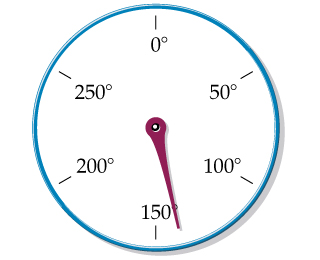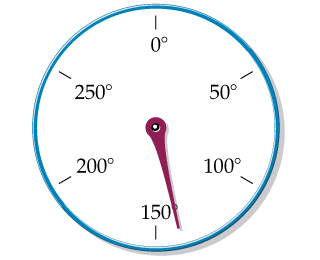# Problem: C. An oven thermometer with a circular scale reading degrees Fahrenheit is shown. What temperature does the scale indicate?How many significant figures are in the measurement in part C?

###### FREE Expert Solution

Given:Estimating, we can assume that the temperature reading is around 140°F.

80% (176 ratings)###### Problem Details

C.

An oven thermometer with a circular scale reading degrees Fahrenheit is shown. What temperature does the scale indicate?How many significant figures are in the measurement in part C?

Frequently Asked Questions

What scientific concept do you need to know in order to solve this problem?

Our tutors have indicated that to solve this problem you will need to apply the Significant Figures: Precision in Measurements concept. You can view video lessons to learn Significant Figures: Precision in Measurements. Or if you need more Significant Figures: Precision in Measurements practice, you can also practice Significant Figures: Precision in Measurements practice problems.